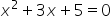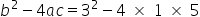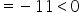Mathematics
Easy

Question

# What is the discriminant value of the equation?

## -11    1    2    Either 1 or 2.    0Hint:

## The correct answer is: -11

### Step 1 of 1:We have given an equationNow,The discriminant value is less than zero, so it has no real solution.

The discriminant is the part of the quadratic formula found within the square root.

### Related Questions to study#### With Turito Foundation.#### Get an Expert Advice From Turito.